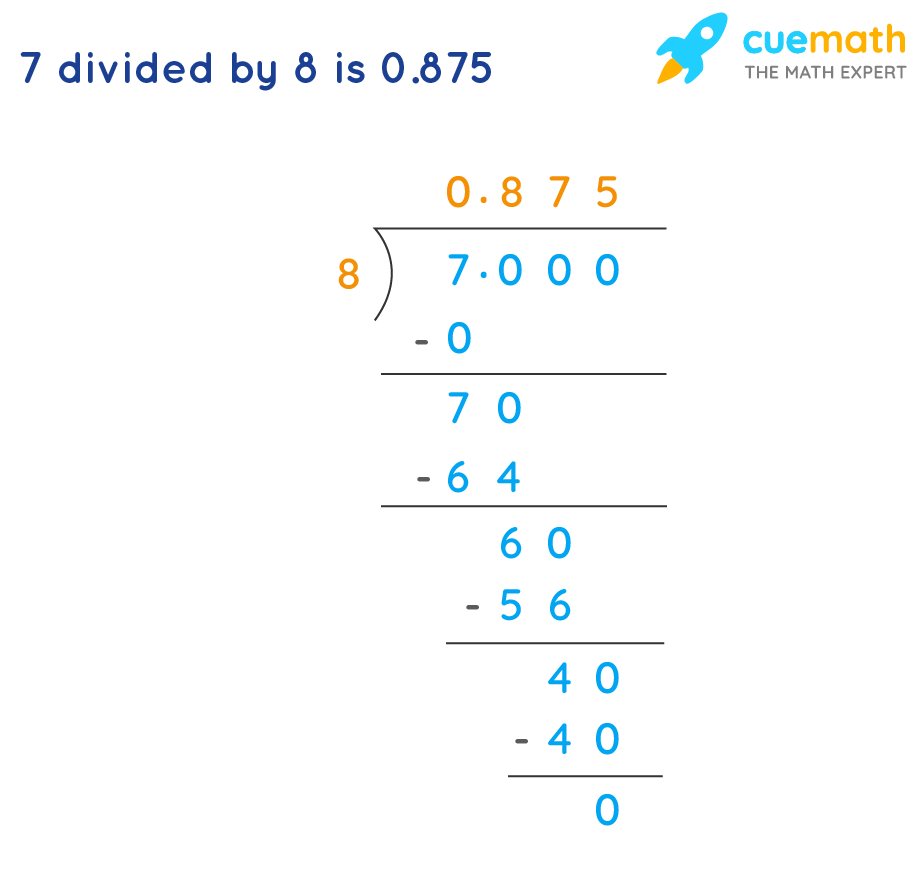# Change a Rational Number to a Decimal

## Question: How do you change a rational number to a decimal?

Numbers that can be expressed in a/b or fraction form is a rational number where a is any integer and b is a non zero integer.

## Answer: In order to change a rational number to a decimal, we divide the numerator with the denominator or can be converted to a decimal by the division method.

We will use the definition of the rational number to explain how to change a rational number to a decimal.

## Explanation:

In order to change a rational number to a decimal, we divide the numerator with the denominator. In order to change a rational number to a decimal, we just convert the number into the form of a fraction. We then divide the numerator with the denominator and find out the exact value of the division.

Consider an example, convert 7/8 to decimal

The below image shows the division method.